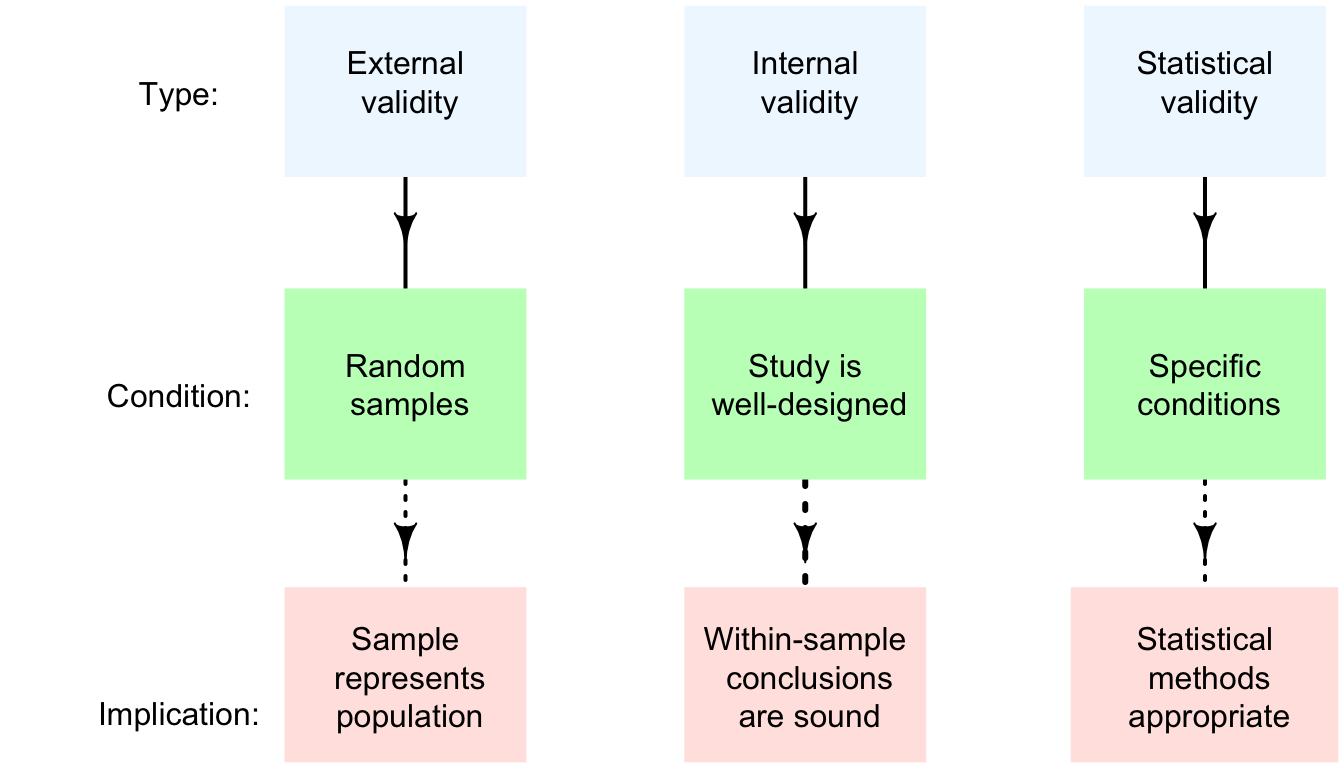## 21.3 Validity and confidence intervals

When constructing confidence intervals, certain statistical validity conditions must be true; these ensure that the sampling distribution is sufficiently close to a normal distribution for the 68–95–99.7 rule rule to apply.

If these conditions are not met, the sampling distribution may not be normally distributed, so the 68–95–99.7 rule (on which the CI is based) maybe inappropriate, so the CI itself may also be inappropriate.

In addition to the statistical validity condition, the internal validity and external validity of the study should be discussed also (Fig. 21.1).

Regarding external validity, all the CI computations in this book assume a simple random sample. If the sample is from a random sampling method, but not from a simple random sample, then methods exist for producing CIs that are externally valid, but are more complicated than those described in this book.

If the sample is a non-random sample, then the CI may be reasonable for the quite specific population that is represented by the sample; however, the sample probably does not represent the more general population that is probably intended.

Externally validity requires that a study is also internally valid. Internal validity can only be discussed if details are known about the study design.FIGURE 21.1: Three types of validities for studies.

In addition, CIs also require that the sample size is less than 10% of the population size; however this is almost always the case.